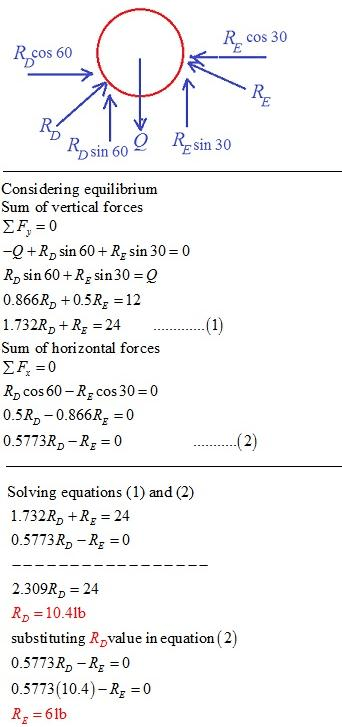# A ball of weight Q = 121b rests in a right-angled trough, as shown in Fig. B. Determine the forces exerted on the sides of the trough at D and E if all surfaces are perfectly smooth.

Question-AnswerCategory: Engineering MechanicsA ball of weight Q = 121b rests in a right-angled trough, as shown in Fig. B. Determine the forces exerted on the sides of the trough at D and E if all surfaces are perfectly smooth.

A ball of weight Q = 121b rests in a right-angled trough, as shown in Fig. B. Determine the forces exerted on the sides of the trough at D and E if all surfaces are perfectly smooth.